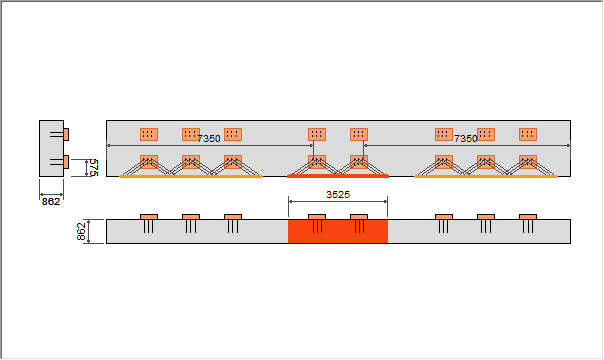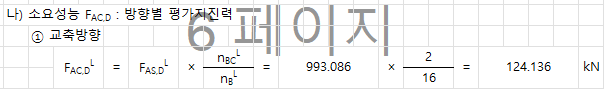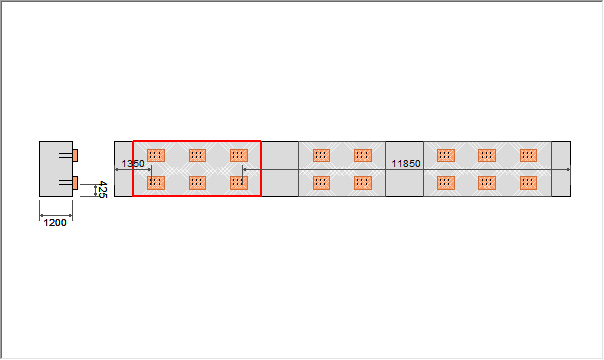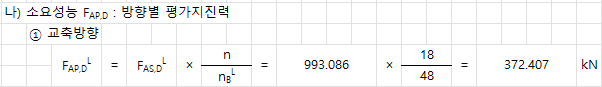Civil Created Edited

# [Civil]The Number of Anchors Used in Support Evaluation for Required Performance Calculation is Inaccurate

## Question

The number of anchors applied to the required performance calculation when performing the support evaluation is incorrect.

In the case of concrete failure, the required performance is calculated by considering the total number of supports resisting the required performance defined based on the bridge criteria, as well as the number of supports applied in the final evaluation.

[Concrete Failure Required Performance] = [Required Performance of the Bridge] x [Number of Supports Applied in Evaluation] / [Number of Supports Resisting Within the Bridge]

Let's consider an example with support placement and resistance groups, as shown in the image below. There are a total of 16 supports arranged, and the number of supports resisting concrete failure is 16. Among them, the evaluation groups are determined as 3/2/3 supports.The concrete failure evaluation is conducted for the 2 supports selected for evaluation. The required performance is calculated by considering all the supports resisting and applying the required performance corresponding to the 2 supports used in the evaluation, as shown in the calculation process below

[Concrete Failure Required Performance] = [Required Performance of the Bridge] x 2/16For pryout failure, it is calculated by considering the total number of anchors resisting the required performance determined on a pier basis and the number of anchors applied in the final evaluation.

[Pryout failure capacity] = [Capacity of pier] x [Number of anchors applied in evaluation]/[Number of resisting anchors in pier]

Let's take the example of anchor placement and resistance grouping as shown in the image below. A total of 16 anchors are placed, and all of them are resisting the failure of the pryout. You can see that the evaluation group is determined to be 6/4/6 anchors.The pryout failure evaluation is performed on the six piers determined to be evaluated, and the required performance is calculated and applied by considering the number of all resisting anchors and the number of resisting anchors placed on the six piers used in the evaluation, as shown in the calculation process below.

[Pryout failure capacity] = [Piers' capacity] x (3x6)/(3x16)0